# Kleene’s Theorem in TOC | Part-1

• Difficulty Level : Easy
• Last Updated : 20 Nov, 2019

A language is said to be regular if it can be represented by using a Finite Automata or if a Regular Expression can be generated for it. This definition leads us to the general definition that; For every Regular Expression corresponding to the language, a Finite Automata can be generated.

For certain expressions like :- (a+b), ab, (a+b)* ; It’s fairly easier to make the Finite Automata by just intuition as shown below. The problem arises when we are provided with a longer Regular Expression. This brings about the need for a systematic approach towards FA generation, which has been put forward by Kleene in Kleene’s Theorem – I

Attention reader! Don’t stop learning now.  Practice GATE exam well before the actual exam with the subject-wise and overall quizzes available in GATE Test Series Course.

Learn all GATE CS concepts with Free Live Classes on our youtube channel.

Kleene’s Theorem-I :

For any Regular Expression r that represents Language L(r), there is a Finite Automata that accepts same language.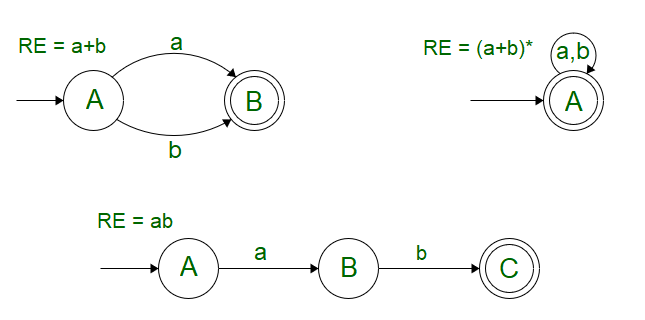To understand Kleene’s Theorem-I, Let’s take in account the basic definition of Regular Expression where we observe that,and a single input symbol “a” can be included in a Regular Language and the corresponding operations that can be performed by the combination of these are:

Say,andbe two regular expressions. Then,

1.+is a regular expression too, whose corresponding language is L() U L()
2..is a regular expression too, whose corresponding language is L().L()
3.* is a regular expression too, whose corresponding language is L()*

We can further use this definition in association with Null Transitions to give rise to a FA by the combination of two or more smaller Finite Automata (each corresponding to a Regular Expression).

Let S accept L = {a} and T accept L = {b}, then R can be represented as a combination of S and T using the provided operations as:

R = S + T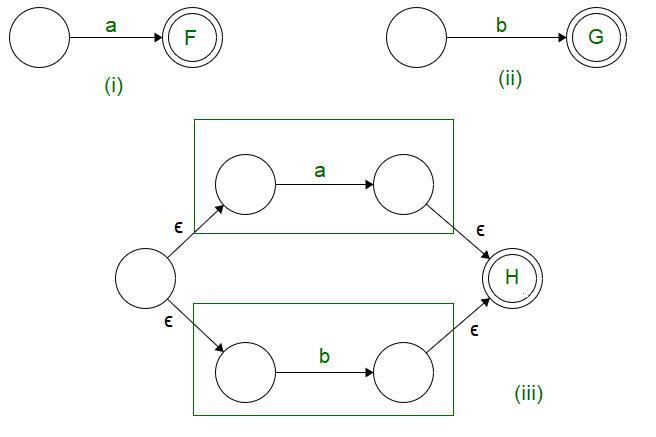We observe that,

1. In case of union operation we can have a new start state, from which, null transition proceeds to the starting state of both the Finite State Machines.
2. The final states of both the Finite Automata’s are converted to intermediate states. The final state is unified into one which can be traversed by null transitions.
R = S.T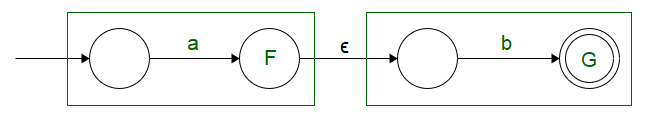We observe that,

1. In case of concatenation operation we can have the same starting state as that of S, the only change occurs in the end state of S, which is converted to an intermediate state followed by a Null Transition.
2. The Null transition is followed by the starting state of T, the final state of T is used as the end state of R.
 R = S*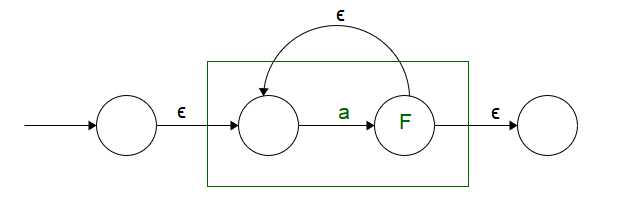We observe that,

1. A new starting state is added, and S has been put as an intermediate state so that self looping condition could be incorporated.
2. Starting and Ending states have been defined separately so that the self looping condition is not disturbed.

Now that we are aware about the general operations. Let’s see how Kleene’s Theorem-I can be used to generate a FA for the given Regular Expression.

Example:
Make a Finite Automata for the expression (ab+a)*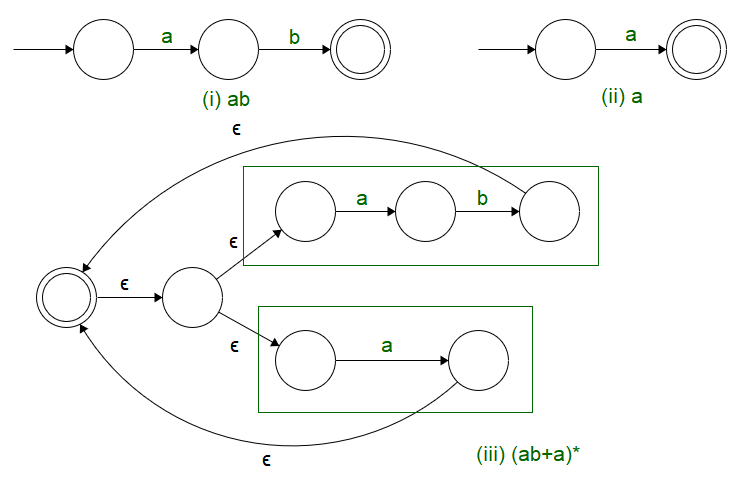We see that using Kleene’s Theorem – I gives a systematic approach towards the generation of a Finite Automata for the provided Regular Expression.

My Personal Notes arrow_drop_up